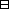## Group Theory and Linear Algebra

Last updated: 24 September 2014

## Lecture 29: Isometries in ${𝔼}^{2}$

Let $s= -1 0 0 1 and rθ= cosθ -sinθ sinθ cosθ$ and let $s$ is reflection in the $y-$axis ${L}_{y}$

${r}_{\theta }$ is rotation in an angle $\theta$ about $0$
${t}_{\gamma }$ is translation by $\gamma$
So $S{O}_{2}\left(ℝ\right)$ is the group of rotations about $0.$
1. Let $L$ be a line in ${ℝ}^{2}.$ Then there exist $c\in ℝ$ and $0\le \theta <\pi$ such that $L= rθt c 0 Ly.$ The reflection in the line $L$ is $sL = rθt c0 st -c0 r-θ.$
2. Let $p\in {ℝ}^{2}$ and $\theta \in \left[0,2\pi \right)$ Then rotation by $\theta$ around $p$ is $rθ,p = tprθt-p.$
3. The $d-$glide reflection in the line $L$ is: translate by a distance $d$ in a line parallel to $L$ and then reflect in $L.$

### Isometries

Let with $d(p,q) = (x1-x2)2+(y1-y2)2 if p= x1 y1 1 and x2 y2 1 .$ An isometry of ${𝔼}^{2}$ is a function $f:{E}^{2}\to {E}^{2}$ such that $d(fp,fq) = d(p,q).$ Note that

• a rotation fixes one point,
• a reflection fixes a line,
• a translation fixes no point.

Let $f:{𝔼}^{2}\to {𝔼}^{2}$ be an isometry. Suppose $\alpha ,p$ are fixed points of $f,$ $fα=α and fβ=β$

Let $p\in {𝔼}^{2}.$ If $p$ is on the line ${L}_{\alpha \beta }$ connecting $\alpha$ and $\beta$ then ${C}_{1}\cap {C}_{2}=\left\{p\right\}.$ So $fp=p$ if $p\in {L}_{\alpha \beta }.$

Thus, if $f:{𝔼}^{2}\to {𝔼}^{2}$ is an isometry and where ${L}_{\alpha \beta }$ is the line connecting $\alpha$ and $\beta .$

If $f:{𝔼}^{2}\to {𝔼}^{2}$ is an isometry and $\gamma ,\alpha ,\beta \in 𝔼$ are such that $\gamma \notin {L}_{\alpha \beta }$ and $\alpha \ne \beta$ and $fα=α, fβ=β and fγ=γ$ then $f$ fixes all of ${𝔼}^{2}.$Proof. $f$ fixes and ${L}_{\beta \gamma }.$ If $p\in {L}_{\alpha \beta }$ and $q\in {L}_{\alpha \gamma }$ then $f$ fixes ${L}_{pq}.$ $Lαβ$ $Lβγ$ $Lpq$ $Lαγ$ $p$ $q$ $α$ $β$ $γ$ $x$ Every point $x\in {𝔼}^{2}$ is on some ${L}_{pq}$ with $p\in {L}_{\alpha \beta }$ and $q\in {L}_{\alpha \beta }$ and so $fx=x.$ So $f={\mathrm{id}}_{{𝔼}^{2}}.$ $\square$

## Notes and References

These are a typed copy of Lecture 29 from a series of handwritten lecture notes for the class Group Theory and Linear Algebra given on October 12, 2011.